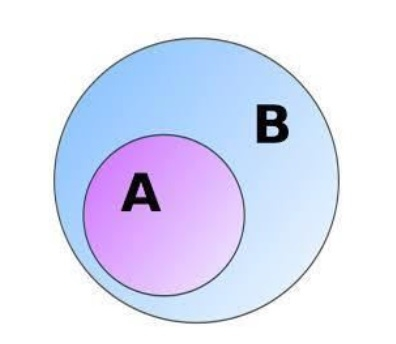# Implement IsNumber() function in Python

This method takes in a string type as input and returns boolean True or False according to whether the entered string is a number or not. To do this we take the help of exception handling by using try and except statement.## Example

Let’s look at some example −

Live Demo

# Implementation of isNumber() function
def isNumber(s):
if(s =='-'):
s=s[1:]
#exception handling
try:
n = int(s)
return True
# catch exception if any error is encountered
except ValueError:
return False
inp1 = "786"
inp2 = "-786"
inp3 = "Tutorialspoint"
print(isNumber(inp1))
print(isNumber(inp2))
print(isNumber(inp3))

## Output

True
True
False

## Conclusion

In this article, we learnt how to implement Implement IsNumber() function in Python 3.x. Or earlier.

Updated on: 29-Aug-2019

70 Views